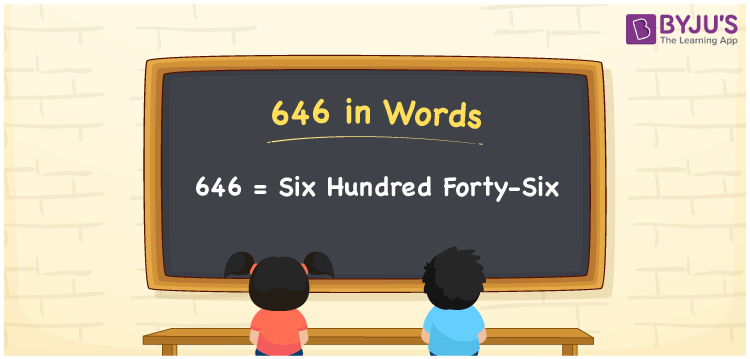# 646 in words

646 in words is written as Six Hundred and Forty Six. 646 represents the count or value. The article on Counting Numbers can give you an idea about count or counting. The number 646 is used in expressions that relate to money, distance, length, year and others. Let us consider an example for 646. ”Can you imagine how the world looked like in the year Six Hundred and Forty Six?”

 646 in words Six Hundred and Forty Six Six Hundred and Forty Six in Numbers 646

## 646 in English Words## How to Write 646 in Words?

We can convert 646 to words using a place value chart. The number 646 has 3 digits, so let’s make a chart that shows the place value up to 3 digits.

 Hundreds Tens Ones 6 4 6

Thus, we can write the expanded form as:

= 6 × Hundred + 4 × Ten + 6 × One

= 6 × 100 + 4 × 10 + 6 × 1

= 646

= Six Hundred and Forty Six.

646 is the natural number that is succeeded by 645 and preceded by 647.

646 in words – Six Hundred and Forty Six.

Is 646 an odd number? – No.

Is 646 an even number? – Yes.

Is 646 a perfect square number? – No.

Is 646 a perfect cube number? – No.

Is 646 a prime number? – No.

Is 646 a composite number? – Yes.

## Solved Example

1. Write the number 646 in expanded form

Solution: 6 × 100 + 4 × 10 + 6 × 1

We can write 646 = 600 + 40 + 6

= 6 × 100 + 4 × 10 + 6 × 1

## Frequently Asked Questions on 646 in words

Q1

### How to write 646 in words?

646 in words is written as Six Hundred and Forty Six.
Q2

### Is 646 a perfect square number?

No. 646 is not a perfect square number.
Q3

### Is 646 a prime number?

No. 646 is not a prime number.